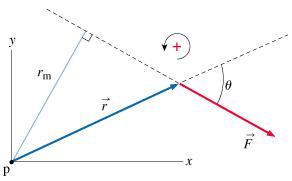# Problem: Find the torque about p due to F. Your answer should correctly express both the magnitude and sign of torque. Express your answer in terms of rm and F or in terms of r, θ, and F.

###### FREE Expert Solution

Torque:

$\overline{){\mathbf{\tau }}{\mathbf{=}}{\mathbf{r}}{\mathbf{f}}}$

rm = r•sin θ

90% (465 ratings)###### Problem DetailsFind the torque about p due to F. Your answer should correctly express both the magnitude and sign of torque. Express your answer in terms of rm and F or in terms of r, θ, and F.